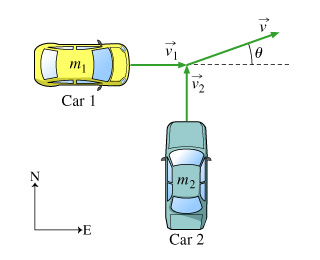# In this problem we will consider the collision of two cars initially moving at right angles

In this problem we will consider the collision of two cars initially moving at right angles. We assume that after the collision the cars stick together and travel off as a single unit. The collision is therefore completely inelastic.

Two cars of masses m1 and m2 collide at an intersection. Before the collision, car 1 was traveling eastward at a speed of v1, and car 2 was traveling northward at a speed of v2. (Figure 1) After the collision, the two cars stick together and travel off in the direction shown.Part A
First, find the magnitude of Vector V, that is, the speed little v of the two-car unit after the collision. Express little v in terms of m1, m2, and the cars’ initial speeds v1 and v2.

Part B
Find the tangent of the angle theta. Express your answer in terms of the momenta of the two cars, p1 and p2.

Part C
Suppose that after the collision, tan=1; in other words, theta is 45 degrees. This means that before the collision:
Choose one:

• The magnitudes of the momenta of the cars were equal.
• The masses of the cars were equal.
• The velocities of the cars were equal.